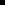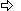#### Sample Alternate Configuration File

The sample configuration file in this section defines the domain configuration for two devices, DEVICE1 and DEVICE2.

Note thatEach Device ID appears as a section heading inside square brackets.The UseTheseDomainSizes variable is set to 1.

This variable must appear in each device section.

the Modbus TCP/IP devcom will auto-size the domains as it always has if any of the following is true.This variable is set to 0.There is no section heading for a device on this port.This .ini file does not exist.

There are 16 domains in a Modbus TCP/IP device. In the alternate configuration file example shown below, you will see each domain's name followed by the default size of that domain. If a domain name is not provided within a device section, the default size is assigned.

[DEVICE1]

UseTheseDomainSizes=1

COILS=65535

DISC INPUTS=65535

INPUT REG.=65535

HOLDING REG.=65535

GEN REF FILE1=0

GEN REF FILE2=0

GEN REF FILE3=0

GEN REF FILE4=0

GEN REF FILE5=0

GEN REF FILE6=0

GEN REF FILE7=0

GEN REF FILE8=0

GEN REF FILE9=0

GEN REF FILE10=0

DP_INPUT REG.=0

DP_HOLDING REG.=0

[DEVICE2]

UseTheseDomainSizes=1

COILS=65535

DISC INPUTS=65535

INPUT REG.=65535

HOLDING REG.=65535

GEN REF FILE1=0

GEN REF FILE2=0

GEN REF FILE3=0

GEN REF FILE4=0

GEN REF FILE5=0

GEN REF FILE6=0

GEN REF FILE7=0

GEN REF FILE8=0

GEN REF FILE9=0

GEN REF FILE10=0

DP_INPUT REG.=0

DP_HOLDING REG.=0

 Step 1.2.2. Entering Required Configuration Data.